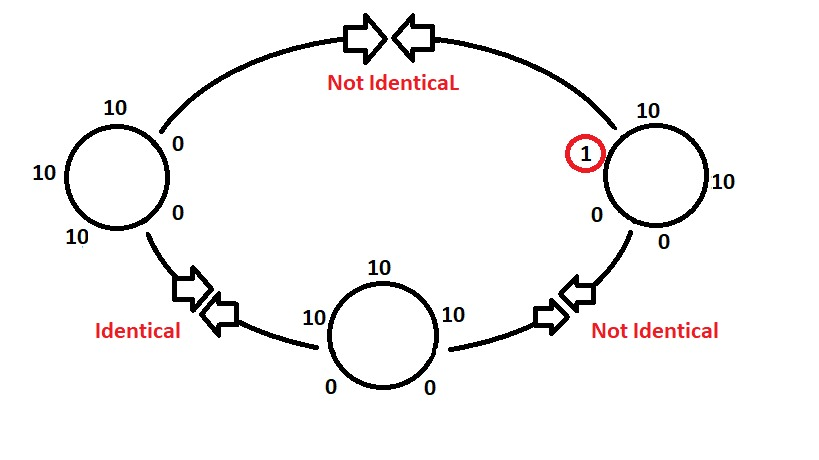Open in App
Not now

# Python | Check whether two lists are circularly identical

• Difficulty Level : Easy
• Last Updated : 29 Dec, 2022

Given two lists, check if they are circularly identical or not.Examples:

```Input : list1 = [10, 10, 0, 0, 10]
list2 = [10, 10, 10, 0, 0]
Output : Yes
Explanation: yes they are circularly identical as when we write the list1
last index to second last index, then we find it is circularly
same with list1
Input : list1 = [10, 10, 10, 0, 0]
list2 = [1, 10, 10, 0, 0]
Output :No```

Method 1 : Using List Traversal

Using traversal, we have to double the given list. Check for any x(0<=n) to any x+n and compare with list2 to see if the list1 and list2 are same, if both are same then the list2 is circularly identical. Using two loops, check this property. The first loop will run from 0 to len(list1) and then check if the index (x to x+n) is same as list2, if yes then return true, else return false. Below is the Python implementation of the above approach:

## Python3

 `# python program to check if two``# lists are circularly identical``# using traversal` `# function to check circularly identical or not``def` `circularly_identical(list1, list2):``    ` `    ``# doubling list``    ``list3 ``=` `list1 ``*` `2``    ` `    ``# traversal in twice of list1``    ``for` `x ``in` `range``(``0``, ``len``(list1)):``        ``z ``=` `0``        ` `        ``# check if list2 == list1 circularly``        ``for` `y ``in` `range``(x, x ``+` `len``(list1)):``            ``if` `list2[z]``=``=` `list3[y]:``                ``z``+``=` `1``            ``else``:``                ``break``            ` `        ``# if all n elements are same circularly``        ``if` `z ``=``=` `len``(list1):``            ``return` `True``    ` `    ``return` `False``        `   `# driver code``list1 ``=` `[``10``, ``10``, ``0``, ``0``, ``10``]``list2 ``=` `[``10``, ``10``, ``10``, ``0``, ``0``]``list3 ``=` `[``1``, ``10``, ``10``, ``0``, ``0``]`  `# check for list 1 and list 2``if``(circularly_identical(list1, list2)):``    ``print``("Yes")``else``:``    ``print``("No")` `# check for list 2 and list 3``if``(circularly_identical(list2, list3)):``    ``print` `("Yes")``else``:``    ``print` `("No")`

Output:

```Yes
No```

Method 2 : Using List Slicing

## Python3

 `# python program to check if two``# lists are circularly identical``# using traversal`` ` `# function to check circularly identical or not``def` `circularly_identical(list1, list2):`` ` `  ``# doubling list``  ``list1.extend(list1)`` ` `  ``# traversal in twice of list1``  ``for` `i ``in` `range``(``len``(list1)):`` ` `    ``# check if sliced list1 is equal to list2``    ``if` `list2 ``=``=` `list1[i: i ``+` `len``(list2)]:`` ` `      ``return` `True``  ``return` `False`  `# driver code``list1 ``=` `[``10``, ``10``, ``0``, ``0``, ``10``]``list2 ``=` `[``10``, ``10``, ``10``, ``0``, ``0``]``list3 ``=` `[``1``, ``10``, ``10``, ``0``, ``0``]`  `# check for list 1 and list 2``if``(circularly_identical(list1, list2)):``    ``print``("Yes")``else``:``    ``print``("No")` `# check for list 2 and list 3``if``(circularly_identical(list2, list3)):``    ``print` `("Yes")``else``:``    ``print` `("No")`

Output:

```Yes
No```

Method 3 : Using map() function

Using Python’s inbuilt function map() we can do this in one single step, where we have to map list2 in a string and then see if it exists in the mapping of twice of list1 (2*list1) in another string. Below is the Python implementation of the above approach:

## Python3

 `# python program to check if two``# lists are circularly identical``# using map function` `# function to check circularly identical or not``def` `circularly_identical(list1, list2):``   ` `    ``return``(``' '``.join(``map``(``str``, list2)) ``in` `' '``.join(``map``(``str``, list1 ``*` `2``)))``    `  `# driver code``list1 ``=` `[``10``, ``10``, ``0``, ``0``, ``10``]``list2 ``=` `[``10``, ``10``, ``10``, ``0``, ``0``]``list3 ``=` `[``1``, ``10``, ``10``, ``0``, ``0``]`  `# check for list 1 and list 2``if` `(circularly_identical(list1, list2)):``    ``print``("Yes")``else``:``    ``print``("No")` `# check for list 2 and list 3``if``(circularly_identical(list2, list3)):``    ``print` `("Yes")``else``:``    ``print` `("No")`

Output:

```Yes
No```

You can use the deque class and its rotate() method to rotate the elements of the first list and check if it is equal to the second list at each rotation.

Here is an example of how to use this approach:

## Python3

 `from` `collections ``import` `deque` `def` `are_circularly_identical(list1, list2):``    ``d ``=` `deque(list1)``    ``for` `_ ``in` `range``(``len``(list1)):``        ``if` `all``(a ``=``=` `b ``for` `a, b ``in` `zip``(d, list2)):``            ``return` `True``        ``d.rotate(``1``)``    ``return` `False` `list1 ``=` `[``10``, ``10``, ``0``, ``0``, ``10``]``list2 ``=` `[``10``, ``10``, ``10``, ``0``, ``0``]``list3 ``=` `[``1``, ``10``, ``10``, ``0``, ``0``]` `print``(are_circularly_identical(list1, list2))  ``# True``print``(are_circularly_identical(list2, list3))  ``# False`

Output

```True
False```

The are_circularly_identical() function takes two lists as input and returns a boolean value indicating whether they are circularly identical or not.

To check for circular identity, the function first creates a deque object from the first list using the deque() function from the collections module. The deque class is a double-ended queue that provides efficient insertion and deletion at both ends and has a rotate() method that rotates the elements of the queue.

The function then uses a for loop to iterate over the range of the length of the first list. On each iteration, it rotates the elements of the deque object by one position using the rotate() method.

After each rotation, the function uses the all() function and a generator expression to check if all elements of the deque object are equal to the corresponding elements of the second list. If this is the case, the function returns True, indicating that the lists are circularly identical. If the deque object is not equal to the second list after any rotation, the function returns False.

For example, when are_circularly_identical() is called with the lists [10, 10, 0, 0, 10] and [10, 10, 10, 0, 0], the deque object is initialized as deque([10, 10, 0, 0, 10]). On the first iteration of the for loop, the rotate() method rotates the elements of the deque object by one position, resulting in deque([10, 0, 0, 10, 10]). The all() function then compares each element of the deque object with the corresponding element of the second list and returns True because they are all equal. The function then returns True as the result.

My Personal Notes arrow_drop_up